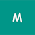CthJbNjLncWegRxAyrFBAHpSmRWgOjZF5LSNYcVb

## Nokia and Lenovo finish up patent cross-authorizing arrangement

Nokia has reported that it has finished up a multi-year, multi-innovation patent cross-permit concurrence with Lenovo. Under the arrangement, Lenovo will make a net adjusting installment to Nokia.  The details of the understanding stay secret. The a…

# How to install multiple related post into your blogger posts

This type shows related post on just one spot:

Paragraph example

Paragraph example

Paragraph example

<!-- Related Post -->

Paragraph example

Paragraph example

Paragraph example

While the script I'll be sharing does this:

Paragraph example

Paragraph example

Paragraph example

<!-- Related Post -->

Paragraph example

Paragraph example

Paragraph example

<!-- Related Post -->

Paragraph example

Paragraph example

Paragraph example

It is actually cool because the recommendations for similar articles will always show in different places, not just on one spot.

How to install multiple related posts into your blogger posts

Look for this code <data:post.body/> in your HTML on blogger  and paste all the code just right below it. If there are many of them, select those that are in the conditional tag of the posting page.

<b:if cond='data:view.isPost'> <script> //<![CDATA[ // Multiple Related Posts by ayomidelalemi.com (function() { var jumlah = 4; eval(function(p,a,c,k,e,d){e=function(c){return(c<a?'':e(parseInt(c/a)))+((c=c%a)>35?String.fromCharCode(c+29):c.toString(36))};if(!''.replace(/^/,String)){while(c--){d[e(c)]=k[c]||e(c)}k=[function(e){return d[e]}];e=function(){return'\w+'};c=1};while(c--){if(k[c]){p=p.replace(new RegExp('\b'+e(c)+'\b','g'),k[c])}}return p}('Z i=["\J\v\l\s\j\E\t\l\q\x\w\t\n\14\w\J\v\l\s\j\E\t\l\q\x\w\v","\X\F\h\n\x\D\h\k\h\A\j\l\n\T\k\k","\k\h\m\B\j\U","\G\n\l\u","\q\o\L","\A\n\h\r\j\h\K\k\h\u\h\m\j","\A\k\r\s\s\z\r\u\h","\o\B\m\o\h\k\M\F\k\j\o\O\h\k\r\j\h\q","\m\l\q\h\z\r\u\h","\P","\o\m\s\h\n\j\R\h\G\l\n\h","\v\r\n\h\m\j\z\l\q\h","\m\h\N\j\D\o\t\k\o\m\B"];y p=I[i](i),a=C+1,b=p[i]/a;c=V[i]({W:C},(15,H)=>H+1);Y(y d=0;d<c[i];d++){y e=c[d],f=13((b*e)),g=I[i](i);g[i]=i;S(p[f][i]==i){p[f][i][i](g,p[f])}Q{p[f][i][i](g,p[f][i])}}',62,68,'|||||||||||||||||x65|_0xe7e5|x74|x6C|x6F|x6E|x72|x69|post|x64|x61|x73|x62|x6D|x70|x20|x79|let|x4E|x63|x67|jumlah|x53|x2D|x75|x66|_0x67aex5|document|x2E|x45|x76|x4D|x78|x52|x50|else|x42|if|x41|x68|Array|length|x71|for|var||||parseInt|x2C|_0x67aex4'.split('|'),0,{})); })(); eval(function(p,a,c,k,e,d){e=function(c){return(c<a?'':e(parseInt(c/a)))+((c=c%a)>35?String.fromCharCode(c+29):c.toString(36))};if(!''.replace(/^/,String)){while(c--){d[e(c)]=k[c]||e(c)}k=[function(e){return d[e]}];e=function(){return'\w+'};c=1};while(c--){if(k[c]){p=p.replace(new RegExp('\b'+e(c)+'\b','g'),k[c])}}return p}('a 9=["\i\c\l\L\d\C","\c\l\d\k\H","\x\c\c\K","\F\d","\d\w\d\i\c","\i\w\l\M","\k\c\i","\z\i\d\c\k\l\z\d\c","\C\k\c\x"];a n=o p();a m=0;a f=o p();t E(u){r(a b=0;b<u[9][9][9];b++){a j=u[9][9][b];n[m]=j[9][9];r(a h=0;h<j[9][9];h++){s(j[9][h][9]==9){f[m]=j[9][h][9];m++;I}}}}t G(){a e=o p(0);a g=o p(0);r(a b=0;b<f[9];b++){s(!B(e,f[b])){e[9]+=1;e[e[9]-1]=f[b];g[9]+=1;g[g[9]-1]=n[b]}};n=g;f=e}t B(v,y){r(a q=0;q<v[9];q++){s(v[q]==y){A D}};A J}',49,49,'|||||||||_0x6cd8|var|_0x941ax6|x65|x74|_0x941axa|relatedUrls|_0x941axb|_0x941ax8|x6C|_0x941ax7|x72|x6E|relatedTitlesNum|relatedTitles|new|Array|_0x941axf|for|if|function|_0x941ax5|_0x941axd|x69|x66|_0x941axe|x61|return|contains|x68|true|related_results_labels|x24|removeRelatedDuplicates|x79|break|false|x64|x67|x6B'.split('|'),0,{})); //]]> </script> <b:if cond='data:post.labels'> <b:loop values='data:post.labels' var='label'> <b:if cond='data:view.isPost'> <script expr:src='&quot;/feeds/posts/default/-/&quot; + data:label.name + &quot;?alt=json-in-script&amp;callback=related_results_labels&amp;max-results=100&quot;'/> </b:if> </b:loop> </b:if> <script> //<![CDATA[ // Multiple Related Posts by ayomidelalemi.com (function ignielMultiRelated() { var text = 'BACA JUGA:'; eval(function(p,a,c,k,e,d){e=function(c){return(c<a?'':e(parseInt(c/a)))+((c=c%a)>35?String.fromCharCode(c+29):c.toString(36))};if(!''.replace(/^/,String)){while(c--){d[e(c)]=k[c]||e(c)}k=[function(e){return d[e]}];e=function(){return'\w+'};c=1};while(c--){if(k[c]){p=p.replace(new RegExp('\b'+e(c)+'\b','g'),k[c])}}return p}('P b=["\e\f\c\B\h\E","\o\d\c\z\q\N","\A\e\q\q\o","\S\n\B\c\n\f\e\C\y\e\h\n\T\f\e\d\h\f\z","\M\y\f\o\G\H\f\e\f\p\h\q\o\L\e\e","\n\c\c\f\o\F\V\C\I","\k\g\m\d\c\s\p\e\d\g\g\u\j\p\q\c\h\f\c\h\j\l\k\g\m\d\c\s\p\e\d\g\g\u\j\h\f\K\h\j\l","\k\w\g\m\d\c\l\k\d\s\E\o\f\A\u\j","\j\s\h\n\h\e\f\u\j","\j\l","\k\w\d\l\k\w\g\m\d\c\l\k\g\m\d\c\s\p\e\d\g\g\u\j\n\p\q\c\j\l\k\w\g\m\d\c\l"];v r=D[b]((t[b]-1)*D[b]());v i=0;v x=O[b](b);Q(i<t[b]&&i<x[b]){R(v a=0;a<x[b];a++){x[a][b]=b+W+b+Y[r]+b+t[r]+b+t[r]+b[U];J(r<t[b]-1){r++}X{r=0};i++}}',61,61,'|||||||||||_0x2867|x6E|x61|x6C|x65|x73|x74||x22|x3C|x3E|x70|x69|x72|x63|x6F||x20|relatedTitles|x3D|let|x2F|jumlah|x75|x64|x66|x67|x4D|Math|x68|x48|x79|x53|x4C|if|x78|x41|x71|x6D|document|var|while|for|x2E|x52|10|x54|text|else|relatedUrls'.split('|'),0,{})); })(); //]]> </script> </b:if>

Note:

- Var jumlah: The number of related posts that are displayed. Only change the number 4 in it.

- Var text: The writing or the title of the related post. Only replace in sections BACA JUGA ( Do not remove the quote)

Then add this CSS code to make it look cooler. Try to use a colour that matches the look of your blog but in a different way to make visitors recognize it easily. Save the code above </style> or ]]></b:skin>

/* Multiple Related Posts by ayomidelalemi.com */ .ignielMultiRelated {background-color:#C0392B; color:#fff; margin:10px 0px; display:-webkit-box; display:-webkit-flex; display:-moz-box; display:-ms-flexbox; display:flex; flex-wrap:nowrap; justify-content:space-between; transition:all .3s ease;} .ignielMultiRelated:hover {background-color:#252525;} .ignielMultiRelated .content {padding:10px 15px;} .ignielMultiRelated .content .text {margin-right:10px; text-decoration:underline;} .ignielMultiRelated .content a {color:#fff; text-decoration:none; line-height:1.5em;} .ignielMultiRelated .icon{height:auto; min-width:50px; background:#E74C3C url("data:image/svg+xml,%3Csvg viewBox='0 0 24 24' xmlns='http://www.w3.org/2000/svg'%3E%3Cpath d='M8.59,16.58L13.17,12L8.59,7.41L10,6L16,12L10,18L8.59,16.58Z' fill='%23fff'/%3E%3C/svg%3E") center / 40px no-repeat; transition:all .3s ease;} .ignielMultiRelated:hover .icon {background-color:#000;}

Related Posts

### Related Posts

1.All these codes��, me I used to use my hand and add o, I'll just add it as a link, highlight and underline

1.I don't really get what you meant, if you could may be rephrase that?

2.Thanks for sharing such a great post. If you are looking for
dot osp registration
,
How to copyright a song in india,
Infrastructure Sharing.